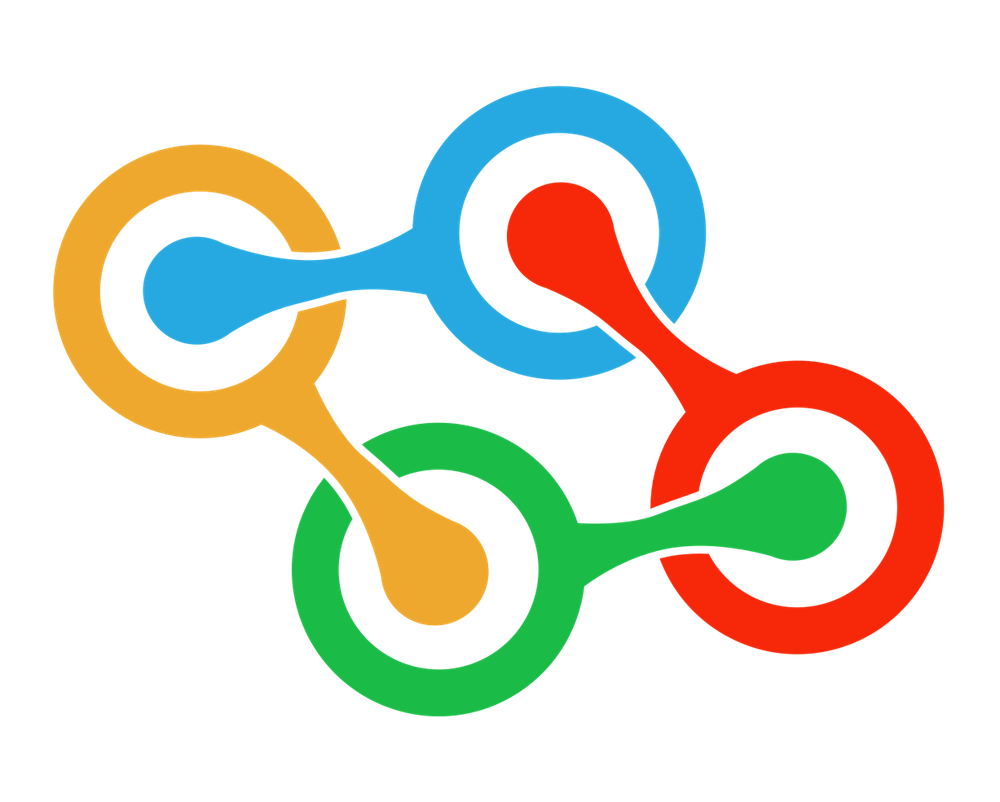# What's in a name?

Why “Matroid”?

At the intersection of Optimization, Linear Algebra, and Graph Theory lies the concept of a Matroid. Matroid theory borrows extensively from the terminology of linear algebra and graph theory, largely because it is the abstraction of various notions of central importance in these fields. Matroids have found applications in geometry, topology, combinatorial optimization, network theory, coding theory, and machine learning.

An introduction to the topics needed to understand Matroids are covered in Stanford’s CME 305 class: Discrete Mathematics and Algorithms taught by Reza Zadeh. Once you have an understanding of these topics, a good introduction to Matroid Theory is given in Michel Goemans’ notes on the topic at MIT.

Machine Learning lies at the heart of the same fields as Matroid Theory, and it is convenient the word “Matroid” sounds much like “Android”. Furthermore, a Matroid is a generalization of a Tensor, and we are using Tensors and TensorFlow heavily. As an Artificial Intelligence company, these coincidences have inspired us to take the name.

Lastly, with the rebirth of Neural Networks and Deep Learning, we feel networks, graphs, and their properties are central to Machine Learning and AI. For this reason, our logo is reminiscent of a Recurrent Neural Network, as well as a Graphic Matroid which is one of the most useful matroids.Despite all the rigor, matroids can be humerous:

A Matroid is a generalization of a Tensor. https://t.co/r44aXx8Ng5 pic.twitter.com/6RSRE4XVX8

— Matroid (@matroid) October 21, 2016